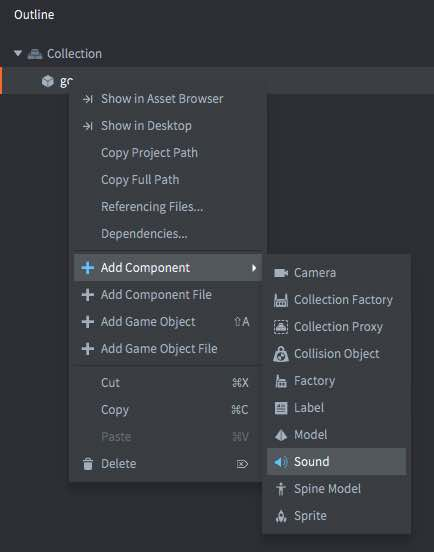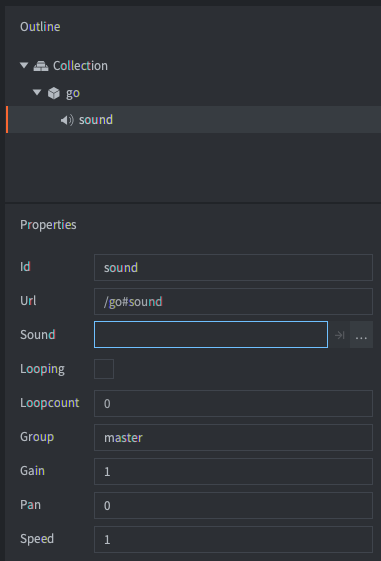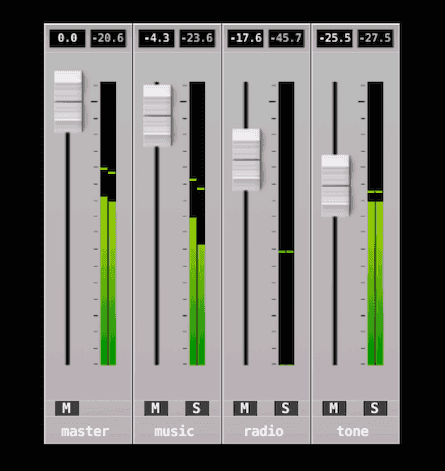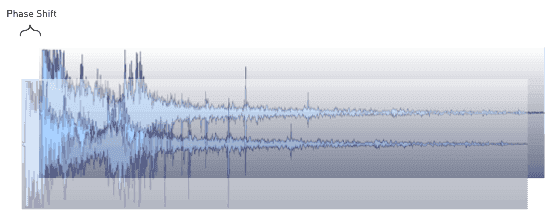# 声音

Defold 支持声音但是不那么强大. 要注意两个概念:

## 创建声音组件Sound

Looping

Loopcount

Group

Gain

Pan

Speed

## 播放声音

sound.play("go#sound", {delay = 1, gain = 0.5, pan = -1.0, speed = 1.25})


## 停止播放声音

sound.stop("go#sound")


## 增益• 声音组件增益属性设置.
• 调用 sound.play() 函数或者直接调用 sound.set_gain() 函数设置的增益.
• 调用 sound.set_group_gain() 函数设置的增益.
• “master” 组上设置的增益. 可以通过调用 sound.set_group_gain(hash("master")) 函数修改设置.

## 声音组

-- 如果 iPhone/Android 设备的播放器正在播放引用, 则所有游戏声音静音
if sound.is_music_playing() then
for i, group_hash in ipairs(sound.get_groups()) do
sound.set_group_gain(group_hash, 0)
end
end$db = 20 \times \log \left( gain \right)$
for i, group_hash in ipairs(sound.get_groups()) do
-- 字符串组名只在调试时可用. 发布后会变成 "unknown_*".
local name = sound.get_group_name(group_hash)
local gain = sound.get_group_gain(group_hash)

-- 转换为分贝.
local db = 20 * math.log10(gain)

-- 得到 RMS (增益均方根). 左右声道分开计算.
local left_rms, right_rms = sound.get_rms(group_hash, 2048 / 65536.0)
left_rmsdb = 20 * math.log10(left_rms)
right_rmsdb = 20 * math.log10(right_rms)

-- 得到峰值. 左右声道分开计算.
left_peak, right_peak = sound.get_peak(group_hash, 2048 * 10 / 65536.0)
left_peakdb = 20 * math.log10(left_peak)
right_peakdb = 20 * math.log10(right_peak)
end

-- 设置主声道增益为 +6 dB (math.pow(10, 6/20)).
sound.set_group_gain("master", 1.995)


## 控制声音-- 在一定 "过滤时间段" 内不允许再次播放同一声音.
local gate_time = 0.3

function init(self)
-- 把计时器保存到一个表里然后每帧计时递减
-- 直到过了 "过滤时间段". 再删除它.
self.sounds = {}
end

function update(self, dt)
-- 计时器递减
for k,_ in pairs(self.sounds) do
self.sounds[k] = self.sounds[k] - dt
if self.sounds[k] < 0 then
self.sounds[k] = nil
end
end
end

function on_message(self, message_id, message, sender)
if message_id == hash("play_gated_sound") then
-- 表里没有才能播放.
if self.sounds[message.soundcomponent] == nil then
-- 保存计时器
self.sounds[message.soundcomponent] = gate_time
-- 播放声音
sound.play(message.soundcomponent, { gain = message.gain })
else
-- 过滤表里有的声音
print("gated " .. message.soundcomponent)
end
end
end


msg.post("/sound_gate#script", "play_gated_sound", { soundcomponent = "/sounds#explosion1", gain = 1.0 })


## 运行时控制

gain

pan

speed

sound

local boom = sys.load_resource("/sounds/boom.wav")
local path = go.get("#sound", "sound")
resource.set_sound(path, boom)


## 相关项目配置

game.project 文件里有些关于声音组件的 设置项目.

Did you spot an error or do you have a suggestion? Please let us know on GitHub!